# Questions on Uncertainties

• TheFallen018
I answered false to this, because in part (ii) the uncertainty in x is larger than the uncertainty in y. The value of s would be smaller.Question 2cIn part (ii), since the value of y is smaller than the uncertainty in x, the value of s is equal to the...I answered false to this, because in part (ii) the uncertainty in x is larger than the uncertainty in y. The value of s would be smaller.Question 2dIn part (ii), since the value of y is smaller than the uncertainty in x, the value of s is equal to the...I answered false to this, because in part (ii) the uncertainty in xf

#### TheFallen018

Member advised to use the formatting template for all homework help requests
Hello,

I've been doing this quiz that is mostly based around uncertainties. I've got a few questions that I've got wrong, and was wondering if someone could give each a brief explanation. There's a few to go over, so if you'd be willing, I would be very grateful.

I've had a crack at these questions, but my understanding seems to be lacking. I could probably keep going on the questions until I get them right, but I don't feel like that would help my understanding much.

Here's the first question. Apparently two of these answers are correct.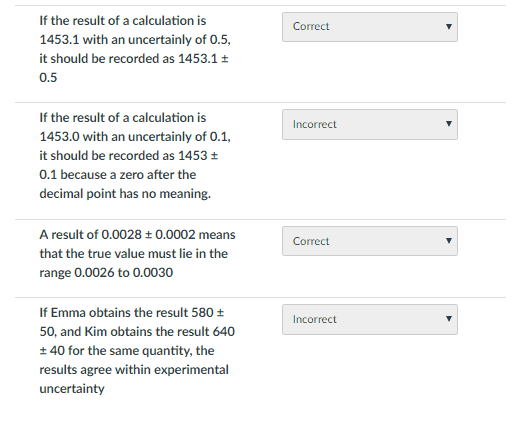Here is question two. Two of these are wrong. I think that part b and c need to be swapped, but I'm not clear on why.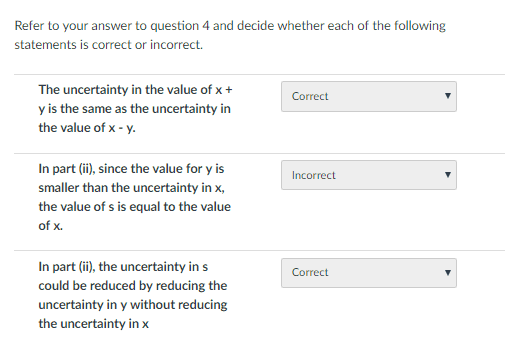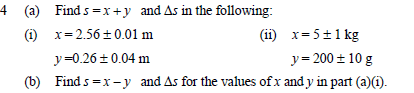The next part has one wrong. I can't tell where the error is, so my understanding of how uncertainties work is clearly missing something here.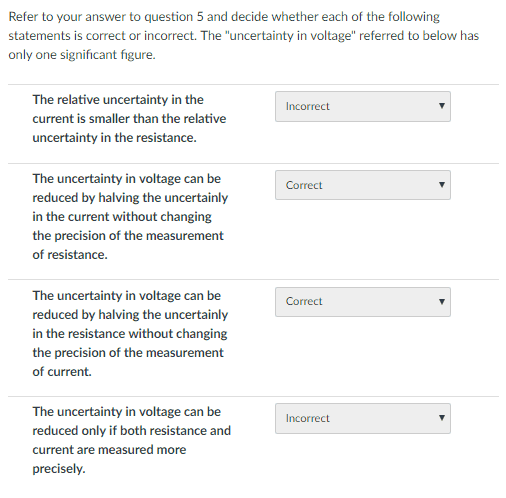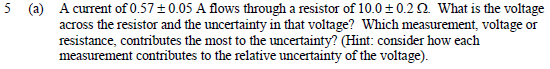This one bothered me, because I can't see how some of the questions make sense, so some explanation on what I did wrong here would go a long way. I measured the standard deviation to be about 0.76, and the standard error in the mean to be about 0.17. The first question seemed strange because with just those measurements the standard deviation was already well below 1m. There are 2 wrong here.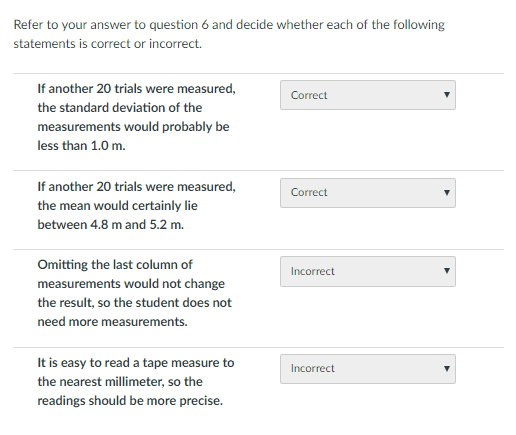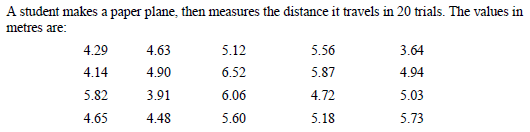Sorry for all of these questions. I really appreciate the time you've taken to read this far.

Thank-you. :)

#### Attachments

To clarify you wish an explanation of why the questions presented are either correct or incorrect?

I've got a few questions that I've got wrong, and was wondering if someone could give each a brief explanation.

The conventions of the homework sections of the forum are that you should not simply ask "Why is this the right answer?" or "Why is this a wrong answer?". Explain your calculations or reasoning for thinking an answer is correct - or incorrect.

Your writing does not clearly state your questions. For example, you write "Here's the first question" and then you quote a question from an exercise. That doesn't explain what your own first question is about the material. What is your own first question?

To clarify you wish an explanation of why the questions presented are either correct or incorrect?
The conventions of the homework sections of the forum are that you should not simply ask "Why is this the right answer?" or "Why is this a wrong answer?". Explain your calculations or reasoning for thinking an answer is correct - or incorrect.

Your writing does not clearly state your questions. For example, you write "Here's the first question" and then you quote a question from an exercise. That doesn't explain what your own first question is about the material. What is your own first question?

Sorry about that. I guess I was trying to keep from writing a small essay. I'll go through my reasoning for each, but I'm sure you'll find my reasoning to be quite flawed. I would like it if you could pick me up on where my reasoning falls short so that I can improve my understanding of these questions.

Question 1a
If the result of a calculation is 1453.1 with an uncertainly of 0.5, it should be recorded as 1453.1 ± 0.5
I answered true to this, because the decimal places match, so this should be correct notation.

Question 1b
If the result of a calculation is 1453.0 with an uncertainly of 0.1, it should be recorded as 1453 ± 0.1 because a zero after the decimal point has no meaning.
I answered false to this, because the decimal place in the value is important as it shows precision. Also, as before, the decimal places need to match.

Question 1c
A result of 0.0028 ± 0.0002 means that the true value must lie in the range 0.0026 to 0.0030
Perhaps this one seemed too straightforward here, and misled me. I answered true to this one. It seemed that the +- should give the values between the range [0.0026,0.0030]

Question 1d
If Emma obtains the result 580 ± 50, and Kim obtains the result 640 ± 40 for the same quantity, the results agree within experimental uncertainty
I answered incorrect to this, as the values for the second one could be much higher for the first. However, upon re-thinking this one, I think it would make sense fot this to be correct, as the values overlap, and the true value would lie within the overlap.

Question 2a
The uncertainty in the value of x + y is the same as the uncertainty in the value of x - y.
I answered true to this, because since uncertainty is +-, it shouldn't matter if we are adding or subtracting. The uncertainty will add either way

Question 2b
In part (ii), since the value for y is smaller than the uncertainty in x, the value of s is equal to the value of x.I answered incorrect to this one, because the uncertainty could be either positive or negative. However adding y to x will result in x being increased positively. Therefore, I considered this to be false.

Question 2c
In part (ii), the uncertainty in s could be reduced by reducing the uncertainty in y without reducing the uncertainty in x
I answered true to this, because adding two numbers with uncertainties should increase the uncertainty. However, if I was incorrect in Question 2b, then I would probably be incorrect in this one too.

Question 3a
The relative uncertainty in the current is smaller than the relative uncertainty in the resistance.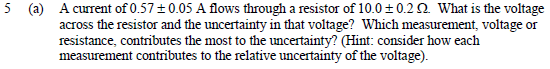I calculated the relative uncertainty to be about 8.8%. This is from ∆x/x = 0.05/0.57 ≈ 0.088. The relative uncertainty for the resistance is 0.2/10=0.02. Therefore the current has a higher relative uncertainty. I answered false to this one.

Question 3b
The uncertainty in voltage can be reduced by halving the uncertainly in the current without changing the precision of the measurement of resistance.
Since voltage is given by V=IR, we need to multiply these together. I answered true to this, because decreasing uncertainty from one side should decrease the uncertainty in the final answer.

Question 3c
The uncertainty in voltage can be reduced by halving the uncertainly in the resistance without changing the precision of the measurement of current.
I answered true to this as well, based on the same logic as the previous question. However, I am guessing that this may be false because the current has the larger uncertainty, and so decreasing uncertainty in the resistance may have a negligible effect. I'm not sure though.

Question 3d
The uncertainty in voltage can be reduced only if both resistance and current are measured more precisely.
I answered false to this, as this contradicted my logic for the previous two questions. Reducing uncertainty in at least the current should decrease the uncertainty in the voltage.

Question 4a
If another 20 trials were measured, the standard deviation of the measurements would probably be less than 1.0 m.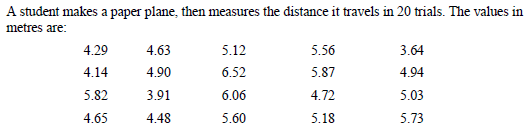I answered true to this, because I calculated the standard deviation to be about 0.76. This was already well under 1 meter, so more measurements would almost certainly keep the standard deviation under a meter.

Question 4b
If another 20 trials were measured, the mean would certainly lie between 4.8 m and 5.2 m.
I answered true to this, because I calculated the mean to be about 5.04m. Since this is between 4.8m and 5.2m I concluded that this should not change much with more measurements, provided they are not wildly different. Therefore, I answered true.

Question 4c
Omitting the last column of measurements would not change the result, so the student does not need more measurements.
I answered false to this, but I'm not sure about it. I answered false because although a small number of measurements should give you a good idea, I would have thought more measurements will always make a more complete picture.

Question 4d
It is easy to read a tape measure to the nearest millimeter, so the readings should be more precise.
I answered false to this, because I thought that the taking averages should more or less cancel out some degree of precision here. Perhaps I'm wrong.

If you've the time, I'd appreciate a few pointers on where my thought process is wrong. Apologies for the long post. Thank you

#### Attachments

Here is question two. Two of these are wrong
Your answers look reasonable to me. One could argue that the value of y is insignificant compared with the error in x, but what if y were 0.9kg±0.01kg?
The next part has one wrong.
Again, I agree with your answers. How have you been taught to calculate uncertainties in products and ratios?

The first question seemed strange because with just those measurements the standard deviation was already well below 1m.
It is not clear whether they mean the s.d. of the new 20 measurements or of all 40 taken together.
Either way, the new s.d. could exceed 1.

Question 1b
If the result of a calculation is 1453.0 with an uncertainly of 0.1, it should be recorded as 1453 ± 0.1 because a zero after the decimal point has no meaning.
I answered false to this, because the decimal place in the value is important as it shows precision. Also, as before, the decimal places need to match.
Perhaps they mean merely that the .0 is unnecessary since you are specifying the uncertainty as ±0.1

Question 1d
If Emma obtains the result 580 ± 50, and Kim obtains the result 640 ± 40 for the same quantity, the results agree within experimental uncertainty
, upon re-thinking this one, I think it would make sense fot this to be correct, as the values overlap, and the true value would lie within the overlap.
Right.

•TheFallen018
Question 4b
If another 20 trials were measured, the mean would certainly lie between 4.8 m and 5.2 m.
I answered true to this, because I calculated the mean to be about 5.04m. Since this is between 4.8m and 5.2m I concluded that this should not change much with more measurements
But it says "certainly"
Question 4c
Omitting the last column of measurements would not change the result, so the student does not need more measurements.
I answered false to this, but I'm not sure about it. I answered false because although a small number of measurements should give you a good idea, I would have thought more measurements will always make a more complete picture.
We are not told why the student is collecting the data, so impossible to say what is needed.
Question 4d
It is easy to read a tape measure to the nearest millimeter, so the readings should be more precise.
I answered false to this, because I thought that the taking averages should more or less cancel out some degree of precision here. P
The readings could be more precise, but whether they should, again, depends on the purpose. Besides, the s.d. of the results is far more than 1mm, so with hindsight it would have been pointless.

•TheFallen018
But it says "certainly"

We are not told why the student is collecting the data, so impossible to say what is needed.

The readings could be more precise, but whether they should, again, depends on the purpose. Besides, the s.d. of the results is far more than 1mm, so with hindsight it would have been pointless.

Thanks for your response. I'm really grateful for the help.

I've been taught to multiply two numbers with uncertainties like this.
(x ± ∆x)*(y ± ∆y) = x*y ± (x*∆y + y*∆x)
Does that look right?

I might have accidentally omitted some detail for question 4. The question is about a student who has made a paper air plane and is trying to determine "the best estimate of its average flight distance with it's error".

I've been taught to multiply two numbers with uncertainties like this.
(x ± ∆x)*(y ± ∆y) = x*y ± (x*∆y + y*∆x)
•